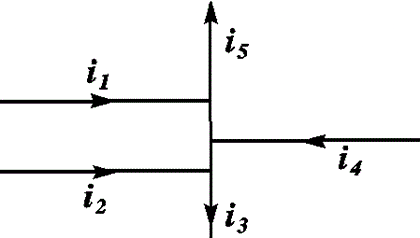# Problem: Calculate the current i5 in the following configuration:i1 = 5.6 Ai2 = 7.9 Ai3 = 8.2 Ai4 = 5.1 A

###### FREE Expert Solution

Kirchhoff's junction rule states that the sum of currents into a junction is equal to the sum of currents out of the junction.

83% (196 ratings)###### Problem Details

Calculate the current i5 in the following configuration:

i1 = 5.6 A
i2 = 7.9 A
i3 = 8.2 A
i4 = 5.1 A